## 5.2.2 Non-MAXWELLian Closure

In the channel of state-of-the-art MOSFET devices, strong gradients of the electric field occur. These highly non-uniform field distributions give rise to distribution functions which deviate significantly from the MAXWELLian distribution. However, the standard energy transport models are based on the assumption of a heated MAXWELLian distribution. Even in a homogeneous semiconductor the distribution function cannot be described properly by a heated MAXWELLian distribution at high field values. Fig. 5.9 shows the distribution function in homogeneous silicon with a bulk doping of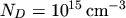. The deviation from the MAXWELLian shape is obvious, which would appear as a straight line due to the logarithmic scale.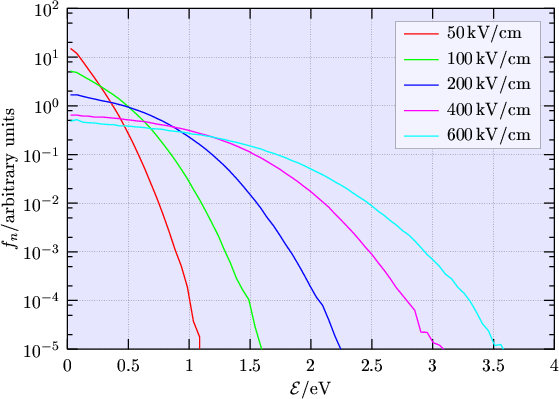The energy flux equation (2.162) contains the moment of fourth order of the distribution function,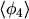: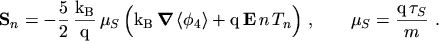(5.36)

To close the set of energy transport equations,needs to be expressed as a function of lower order moments. Assuming a MAXWELLian distribution function gives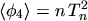, whereas a non-MAXWELLian distribution function can be characterized by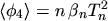, using a non-MAXWELLian parameter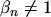.

Regions of the channel where the high energy tail is underpopulated (points B, C, D, E in Fig. 5.6, Region II in Fig. 5.8) are characterized by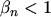.

The non-MAXWELLian effects turned out to be important for SOI simulations. It is believed that standard energy transport SOI simulations yield anomalous results mainly because these effects are neglected. The problem is that in the energy transport framework there is no information about the non-MAXWELLian parameter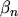. In Chapter 6, several attempts are made to estimate this additional information.

M. Gritsch: Numerical Modeling of Silicon-on-Insulator MOSFETs PDF